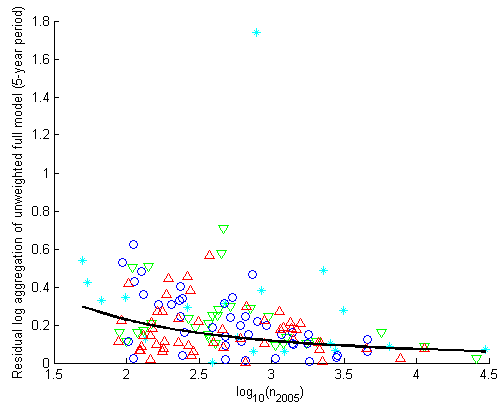Ecological Archives E093-137-A6

Anton J. Flügge, Sofia C. Olhede, and David J. Murrell. 2012. The memory of spatial patterns: changes in local abundance and aggregation in a tropical forest. Ecology 93:1540–1549. http://dx.doi.org/10.1890/11-1004.1

Appendix F. Description of the estimation procedure for the weights used in the weighted regression models.

A plot of the residuals of the linear regression model using current abundance and change of abundance between 2000 and 2005 versus current abundance n2005,i (see Fig. F1) indicates that the variance of the residuals depends on n2005,i. Empirically we model the variance as:

var{ε2005,i} = a2(logn2005,i)2b

This implies that:

log|ε2005,i| = loga + b log |logn2005,i| + v2005,i

Where v2005,i has constant mean and variance (under some circumstances the mean is zero, if it is not, there is no effects of this, as we shall subsequently use weighted least squares). We fit a and b using least squares, and then use weighted regression with this fitted model, with a weighting function given by:

wi = â-2(logn2005,i)-2b

One outlier observation is ignored in this fit.Fig. F1. The relationship between local abundance and the residuals of the linear regression model for all 143 species from BCI that meet our selection criteria (top-canopy trees Δ; middle canopy trees O; understory trees ; shrubs *). The black line shows the estimated standard deviation wi0.5 of the error that was used for the weighted regression in the our analysis.